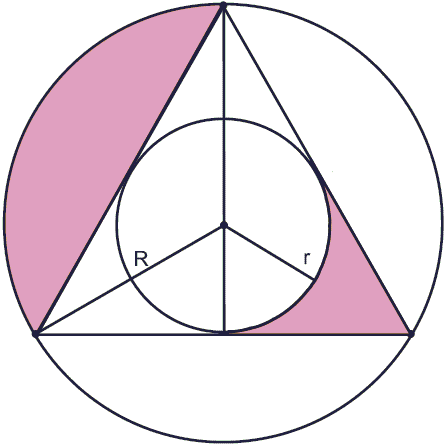# Pairs of Areas in Equilateral Triangle

Prove that the sum of the shaded areas in the figure below is equal to the area of the incircle of the equilateral triangle.Solution

### References

1. B. J. McCartin, An Elementary Property of the Equilateral Triangle: A Tale of Two Proofs, International Mathematical Forum, Vol. 6, 2011, no. 14, 699 - 701Prove that the sum of the shaded areas in the figure below is equal to the area of the incircle of the equilateral triangle.### Solution

One solution is based on the following observation. There are three pairs of regions whose total area we seek to determine. The area of the circumcircle of the given equilateral triangle is thus split into three pairs of areas in question and the incircle.Let the area in question be S, AR = πR² the area of the circumcircle, and Ar = πr² the area of the incircle.

3S + Ar = AR.

In the equilateral triangle R = 2r. This is so because, in an equilateral triangle, the same lines serve as altitudes, medians, angle and perpendicular bisectors; while the same point serves as the centroid, incenter, and circumcenter. The centroid cuts the median in ratio 2:1. The "2" part is the circumradius, the "1" part is the inradius. Therefore,

3S = 4Ar - Ar = 3Ar,

and, finally, S = Ar, as required.• Equilateral Triangles on Sides of a Quadrilateral
• Euler Line Cuts Off Equilateral Triangle
• Four Incircles in Equilateral Triangle
• Problem in Equilateral Triangle
• Problem in Equilateral Triangle II
• Sum of Squares in Equilateral Triangle
• Triangle Classification
• Isoperimetric Property of Equilateral Triangles
• Maximum Area Property of Equilateral Triangles
• Angle Trisectors on Circumcircle
• Equilateral Triangles On Sides of a Parallelogram
• Pompeiu's Theorem
• Circle of Apollonius in Equilateral Triangle
•